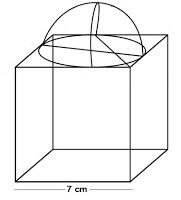# A cubical block of side 7 cm is surmounted by a hemisphere. What is the greatest diameter the hemisphere can have? Find the surface area of the solid.

It is given that each side of cube is 7 cm. So, the radius will be 7/2 cm.We know that,

The total surface area of solid (TSA) = surface area of cubical block + CSA of hemisphere – Area of base of hemisphere

Hence,

TSA of solid = 6×(side)2 + 2πr2 – πr2

= 6 × (side)2 + πr2

= 6 x (7)2 + (22/7) x (7/2) x (7/2)

= 6 x 49 + (77/2)

On further calculation, we get,

= 294 + 38.5

= 332.5 cm2

Therefore, the surface area of the solid is 332.5 cm2